$$\newcommand{\id}{\mathrm{id}}$$ $$\newcommand{\Span}{\mathrm{span}}$$ $$\newcommand{\kernel}{\mathrm{null}\,}$$ $$\newcommand{\range}{\mathrm{range}\,}$$ $$\newcommand{\RealPart}{\mathrm{Re}}$$ $$\newcommand{\ImaginaryPart}{\mathrm{Im}}$$ $$\newcommand{\Argument}{\mathrm{Arg}}$$ $$\newcommand{\norm}{\| #1 \|}$$ $$\newcommand{\inner}{\langle #1, #2 \rangle}$$ $$\newcommand{\Span}{\mathrm{span}}$$

# 1.2: Fractions

$$\newcommand{\vecs}{\overset { \rightharpoonup} {\mathbf{#1}} }$$ $$\newcommand{\vecd}{\overset{-\!-\!\rightharpoonup}{\vphantom{a}\smash {#1}}}$$$$\newcommand{\id}{\mathrm{id}}$$ $$\newcommand{\Span}{\mathrm{span}}$$ $$\newcommand{\kernel}{\mathrm{null}\,}$$ $$\newcommand{\range}{\mathrm{range}\,}$$ $$\newcommand{\RealPart}{\mathrm{Re}}$$ $$\newcommand{\ImaginaryPart}{\mathrm{Im}}$$ $$\newcommand{\Argument}{\mathrm{Arg}}$$ $$\newcommand{\norm}{\| #1 \|}$$ $$\newcommand{\inner}{\langle #1, #2 \rangle}$$ $$\newcommand{\Span}{\mathrm{span}}$$ $$\newcommand{\id}{\mathrm{id}}$$ $$\newcommand{\Span}{\mathrm{span}}$$ $$\newcommand{\kernel}{\mathrm{null}\,}$$ $$\newcommand{\range}{\mathrm{range}\,}$$ $$\newcommand{\RealPart}{\mathrm{Re}}$$ $$\newcommand{\ImaginaryPart}{\mathrm{Im}}$$ $$\newcommand{\Argument}{\mathrm{Arg}}$$ $$\newcommand{\norm}{\| #1 \|}$$ $$\newcommand{\inner}{\langle #1, #2 \rangle}$$ $$\newcommand{\Span}{\mathrm{span}}$$

Learning Objectives

• Find the common denominator of two or more fractions
• Use the common denominator to add or subtract fractions
• Simplify a fraction to its lowest terms
• Multiply fractions
• Multiply two or more fractions
• Multiply a fraction by a whole number
• Divide fractions
• Find the reciprocal of a number
• Divide a fraction by a whole number
• Divide a fraction by a fraction

## Introduction

Before we get started, here is some important terminology that will help you understand the concepts about working with fractions in this section.

• product: the result of multiplication
• factor: something being multiplied – for $$3 \cdot 2 = 6$$ , both 3 and 2 are factors of 6
• numerator: the top part of a fraction – the numerator in the fraction $$\frac{2}{3}$$ is 2
• denominator: the bottom part of a fraction – the denominator in the fraction $$\frac{2}{3}$$ is 3

Many different words are used by math textbooks and teachers to provide students with instructions on what they are to do with a given problem. For example, you may see instructions such as “Find” or “Simplify” in the example in this module. It is important to understand what these words mean so you can successfully work through the problems in this course. Here is a short list of the words you may see that can help you know how to work through the problems in this module.

Instruction Interpretation
Find Perform the indicated mathematical operations which may include addition, subtraction, multiplication, division.
Simplify 1) Perform the indicated mathematical operations including addition, subtraction, multiplication, division

2) Write a mathematical statement in smallest terms so there are no other mathematical operations that can be performed—often found in problems related to fractions and the order of operations

Evaluate Perform the indicated mathematical operations including addition, subtraction, multiplication, division
Reduce Write a mathematical statement in smallest or lowest terms so there are no other mathematical operations that can be performed—often found in problems related to fractions or division

When you need to add or subtract fractions, you will need to first make sure that the fractions have the same denominator. The denominator tells you how many pieces the whole has been broken into, and the numerator tells you how many of those pieces you are using.

The “parts of a whole” concept can be modeled with pizzas and pizza slices. For example, imagine a pizza is cut into 4 pieces, and someone takes 1 piece. Now, $$\frac{3}{4}$$ remains. Note that both of these fractions have a denominator of 4, which refers to the number of slices the whole pizza has been cut into. What if you have another pizza that had been cut into 8 equal parts and 3 of those parts were gone, leaving $$\frac{5}{8}$$?How can you describe the total amount of pizza that is left with one number rather than two different fractions? You need a common denominator, technically called the least common multiple. Remember that if a number is a multiple of another, you can divide them and have no remainder.

One way to find the least common multiple of two or more numbers is to first multiply each by 1, 2, 3, 4, etc. For example, find the least common multiple of 2 and 5.

 First, list all the multiples of 2: Then list all the multiples of 5: $$5\cdot 1 = 5$$ $$5\cdot 2 = 10$$ $$5\cdot 3 = 15$$ $$5\cdot 4 = 20$$ $$5\cdot 5 = 25$$

The smallest multiple they have in common will be the common denominator for the two!

### Example

Describe the amount of pizza left using common terms.

[hidden-answer a=”155500″]Rewrite the fractions $$\frac{5}{8}$$ as fractions with a least common denominator.

Find the least common multiple of the denominators. This is the least common denominator.

Multiples of 4: 4, 8, 12, 16

Multiples of 8: 8, 16, 24

The least common denominator is 8—the smallest multiple they have in common.

Rewrite $$\frac{3}{4}$$ with a denominator of 8. You have to multiply both the top and bottom by 2 so you don’t change the relationship between them.

$$\frac{3}{4}\cdot \frac{2}{2}=\frac{6}{8}$$

We don’t need to rewrite $$\frac{5}{8}$$ since it already has the common denominator.

Both $$\frac{5}{8}$$ have the same denominator, and you can describe how much pizza is left with common terms.

To add fractions with unlike denominators, first rewrite them with like denominators. Then, you know what to do! The steps are shown below.

### Adding Fractions with Unlike Denominators

1. Find a common denominator.
2. Rewrite each fraction using the common denominator.
3. Now that the fractions have a common denominator, you can add the numerators.
4. Simplify by canceling out all common factors in the numerator and denominator.

### Simplifying a Fraction

Often, if the answer to a problem is a fraction, you will be asked to write it in lowest terms. This is a common convention used in mathematics, similar to starting a sentence with a capital letter and ending it with a period. In this course, we will not go into great detail about methods for reducing fractions because there are many. The process of simplifying a fraction is often called reducing the fraction. We can simplify by canceling (dividing) the common factors in a fraction’s numerator and denominator. We can do this because a fraction represents division.

For example, to simplify $$\frac{6}{9}$$ you can rewrite 6 and 9 using the smallest factors possible as follows:

$$\frac{6}{9}=\frac{2\cdot3}{3\cdot3}$$

Since there is a 3 in both the numerator and denominator, and fractions can be considered division, we can divide the 3 in the top by the 3 in the bottom to reduce to 1.

$$\frac{6}{9}=\frac{2\cdot\cancel{3}}{3\cdot\cancel{3}}=\frac{2\cdot1}{3}=\frac{2}{3}$$

Rewriting fractions with the smallest factors possible is often called prime factorization.

In the next example you are shown how to add two fractions with different denominators, then simplify the answer.

### Example

Add $$\frac{2}{3}+\frac{1}{5}$$. Simplify the answer.

[hidden-answer a=”797488″]Since the denominators are not alike, find a common denominator by multiplying the denominators.

$$3\cdot5=15$$

Rewrite each fraction with a denominator of 15.

$$\begin{array}{c}\frac{2}{3}\cdot \frac{5}{5}=\frac{10}{15}\\\\\frac{1}{5}\cdot \frac{3}{3}=\frac{3}{15}\end{array}$$

Add the fractions by adding the numerators and keeping the denominator the same. Make sure the fraction cannot be simplified.

$$\frac{10}{15}+\frac{3}{15}=\frac{13}{15}$$

$$\frac{2}{3}+\frac{1}{5}=\frac{13}{15}$$

You can find a common denominator by finding the common multiples of the denominators. The least common multiple is the easiest to use.

### Example

Add $$\frac{3}{7}+\frac{2}{21}$$. Simplify the answer.

[hidden-answer a=”520906″]Since the denominators are not alike, find the least common denominator by finding the least common multiple (LCM) of 7 and 21.

Multiples of 7: 7, 14, 21

Multiples of 21: 21

Rewrite each fraction with a denominator of 21.

$$\begin{array}{c}\frac{3}{7}\cdot \frac{3}{3}=\frac{9}{21}\\\\\frac{2}{21}\end{array}$$

Add the fractions by adding the numerators and keeping the denominator the same. Make sure the fraction cannot be simplified.

$$\frac{9}{21}+\frac{2}{21}=\frac{11}{21}$$

$$\frac{3}{7}+\frac{2}{21}=\frac{11}{21}$$

In the following video you will see an example of how to add two fractions with different denominators.A YouTube element has been excluded from this version of the text. You can view it online here: pb.libretexts.org/ba/?p=34

You can also add more than two fractions as long as you first find a common denominator for all of them. An example of a sum of three fractions is shown below. In this example, you will use the prime factorization method to find the LCM.

Add $$\frac{3}{4}+\frac{1}{6}+\frac{5}{8}$$. Simplify the answer and write as a mixed number.

What makes this example different than the previous ones? Use the box below to write down a few thoughts about how you would add three fractions with different denominators together.

[practice-area rows=”2″][/practice-area]

[hidden-answer a=”680977″]Since the denominators are not alike, find the least common denominator by finding the least common multiple (LCM) of 4, 6, and 8.

$$4=2\cdot2\\6=3\cdot2\\8=2\cdot2\cdot2\\\text{LCM}:\,\,2\cdot2\cdot2\cdot3=24$$

Rewrite each fraction with a denominator of 24.

$$\begin{array}{c}\frac{3}{4}\cdot \frac{6}{6}=\frac{18}{24}\\\\\frac{1}{6}\cdot \frac{4}{4}=\frac{4}{24}\\\\\frac{5}{8}\cdot \frac{3}{3}=\frac{15}{24}\end{array}$$

Add the fractions by adding the numerators and keeping the denominator the same.

$$\frac{18}{24}+\frac{4}{24}+\frac{15}{24}=\frac{37}{24}$$

Write the improper fraction as a mixed number and simplify the fraction.

$$\frac{37}{24}=1\,\,\frac{13}{24}$$

$$\frac{3}{4}+\frac{1}{6}+\frac{5}{8}=1\frac{13}{24}$$

### Subtracting Fractions

When you subtract fractions, you must think about whether they have a common denominator, just like with adding fractions. Below are some examples of subtracting fractions whose denominators are not alike.

### Example

Subtract $$\frac{1}{5}-\frac{1}{6}$$. Simplify the answer.

[hidden-answer a=”155692″]The fractions have unlike denominators, so you need to find a common denominator. Recall that a common denominator can be found by multiplying the two denominators together.

$$5\cdot6=30$$

Rewrite each fraction as an equivalent fraction with a denominator of 30.

$$\begin{array}{c}\frac{1}{5}\cdot \frac{6}{6}=\frac{6}{30}\\\\\frac{1}{6}\cdot \frac{5}{5}=\frac{5}{30}\end{array}$$

Subtract the numerators. Simplify the answer if needed.

$$\frac{6}{30}-\frac{5}{30}=\frac{1}{30}$$

$$\frac{1}{5}-\frac{1}{6}=\frac{1}{30}$$

The example below shows how to use multiples to find the least common multiple, which will be the least common denominator.

### Example

Subtract $$\frac{5}{6}-\frac{1}{4}$$. Simplify the answer.

[hidden-answer a=”984596″]Find the least common multiple of the denominators—this is the least common denominator.

Multiples of 6: 6, 12, 18, 24

Multiples of 4: 4, 8 12, 16, 20

12 is the least common multiple of 6 and 4.

Rewrite each fraction with a denominator of 12.

$$\begin{array}{c}\frac{5}{6}\cdot \frac{2}{2}=\frac{10}{12}\\\\\frac{1}{4}\cdot \frac{3}{3}=\frac{3}{12}\end{array}$$

Subtract the fractions. Simplify the answer if needed.

$$\frac{10}{12}-\frac{3}{12}=\frac{7}{12}$$

$$\frac{5}{6}-\frac{1}{4}=\frac{7}{12}$$

In the following video you will see an example of how to subtract fractions with unlike denominators.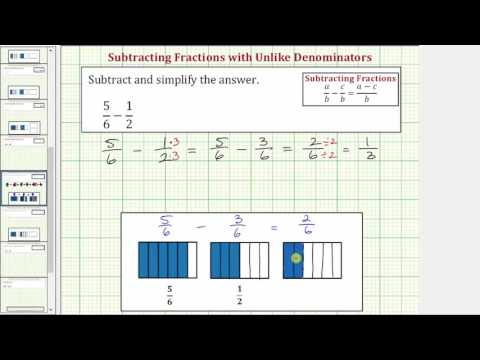A YouTube element has been excluded from this version of the text. You can view it online here: pb.libretexts.org/ba/?p=34

## Multiply Fractions

Just as you add, subtract, multiply, and divide when working with whole numbers, you also use these operations when working with fractions. There are many times when it is necessary to multiply fractions. A model may help you understand multiplication of fractions.

When you multiply a fraction by a fraction, you are finding a “fraction of a fraction.” Suppose you have $$\frac{1}{2}$$ of the $$\frac{3}{4}$$:By dividing each fourth in half, you can divide the candy bar into eighths.Then, choose half of those to get $$\frac{3}{8}$$.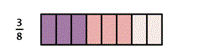In both of the above cases, to find the answer, you can multiply the numerators together and the denominators together.

### Multiplying Two Fractions

$$\frac{a}{b}\cdot \frac{c}{d}=\frac{a\cdot c}{b\cdot d}=\frac{\text{product of the numerators}}{\text{product of the denominators}}$$

### Multiplying More Than Two Fractions

$$\frac{a}{b}\cdot \frac{c}{d}\cdot \frac{e}{f}=\frac{a\cdot c\cdot e}{b\cdot d\cdot f}$$

### Example

Multiply $$\frac{2}{3}\cdot \frac{4}{5}$$.

[hidden-answer a=”368042″]Multiply the numerators and multiply the denominators.

$$\frac{2\cdot 4}{3\cdot 5}$$

Simplify, if possible. This fraction is already in lowest terms.

$$\frac{8}{15}$$

$$\frac{8}{15}$$

To review: if a fraction has common factors in the numerator and denominator, we can reduce the fraction to its simplified form by removing the common factors.

For example,

• Given $$\frac{8}{15}$$ is simplified because there are no common factors of 8 and 15.
• Given $$\frac{10}{15}$$ is not simplified because 5 is a common factor of 10 and 15.

You can simplify first, before you multiply two fractions, to make your work easier. This allows you to work with smaller numbers when you multiply.

In the following video you will see an example of how to multiply two fractions, then simplify the answer.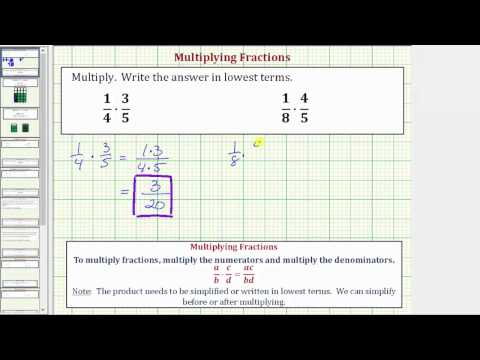A YouTube element has been excluded from this version of the text. You can view it online here: pb.libretexts.org/ba/?p=34

Multiply $$\frac{2}{3}\cdot \frac{1}{4}\cdot\frac{3}{5}$$. Simplify the answer.

What makes this example different than the previous ones? Use the box below to write down a few thoughts about how you would multiply three fractions together.

[practice-area rows=”2″][/practice-area]

[hidden-answer a=”385641″]Multiply the numerators and multiply the denominators.

$$\frac{2\cdot 1\cdot 3}{3\cdot 4\cdot 5}$$

Simplify first by canceling (dividing) the common factors of 3 and 2. 3 divided by 3 is 1, and 2 divided by 2 is 1.

$$\begin{array}{c}\frac{2\cdot 1\cdot3}{3\cdot (2\cdot 2)\cdot 5}\\\frac{\cancel{2}\cdot 1\cdot\cancel{3}}{\cancel{3}\cdot (\cancel{2}\cdot 2)\cdot 5}\\\frac{1}{10}\end{array}$$

$$\frac{1}{10}$$

## Divide Fractions

There are times when you need to use division to solve a problem. For example, if painting one coat of paint on the walls of a room requires 3 quarts of paint and you have a bucket that contains 6 quarts of paint, how many coats of paint can you paint on the walls? You divide 6 by 3 for an answer of 2 coats. There will also be times when you need to divide by a fraction. Suppose painting a closet with one coat only required $$\frac{1}{2}$$.

Before we begin dividing fractions, let’s cover some important terminology.

• reciprocal: two fractions are reciprocals if their product is 1 (Don’t worry; we will show you examples of what this means.)
• quotient: the result of division

Dividing fractions requires using the reciprocal of a number or fraction. If you multiply two numbers together and get 1 as a result, then the two numbers are reciprocals. Here are some examples of reciprocals:

Original number Reciprocal Product
$$\frac{4}{3}$$ $$\frac{1}{2}$$ $$\frac{1}{2}\cdot\frac{2}{1}=\frac{1\cdot}{2\cdot1}=\frac{2}{2}=1$$
$$\frac{1}{3}$$ $$2\frac{1}{3}=\frac{7}{3}$$ $$\frac{7}{3}\cdot\frac{3}{7}=\frac{7\cdot3}{3\cdot7}=\frac{21}{21}=1$$

Sometimes we call the reciprocal the “flip” of the other number: flip $$\frac{5}{2}$$.

## Division by Zero

You know what it means to divide by 2 or divide by 10, but what does it mean to divide a quantity by 0? Is this even possible? Can you divide 0 by a number? Consider the fraction

$$\frac{0}{8}$$

We can read it as, “zero divided by eight.” Since multiplication is the inverse of division, we could rewrite this as a multiplication problem.

$$\text{?}\cdot{8}=0$$.

We can infer that the unknown must be 0 since that is the only number that will give a result of 0 when it is multiplied by 8.

Now let’s consider the reciprocal of $$\frac{8}{0}$$. If we rewrite this as a multiplication problem, we will have

$$\text{?}\cdot{0}=8$$.

This doesn’t make any sense. There are no numbers that you can multiply by zero to get a result of 8. The reciprocal of $$\frac{8}{0}$$ is undefined, and in fact, all division by zero is undefined.

$$\frac{0}{a}$$ will always be undefined.

## Divide a Fraction by a Whole Number

When you divide by a whole number, you are multiplying by the reciprocal. In the painting example where you need 3 quarts of paint for a coat and have 6 quarts of paint, you can find the total number of coats that can be painted by dividing 6 by 3, $$\frac{1}{3}$$, so the multiplication problem becomes

$$\frac{6}{1}\cdot \frac{1}{3}=\frac{6}{3}=2$$.

### Dividing is Multiplying by the Reciprocal

For all division, you can turn the operation into multiplication by using the reciprocal. Dividing is the same as multiplying by the reciprocal.

The same idea will work when the divisor (the thing being divided) is a fraction. If you have $$\frac{1}{5}$$ of the available candy:

$$\frac{1}{5}\text{ of }\frac{3}{4}=\frac{1}{5}\cdot \frac{3}{4}=\frac{3}{20}$$

Each person gets $$\frac{3}{20}$$ of a whole candy bar.

If you have a recipe that needs to be divided in half, you can divide each ingredient by 2, or you can multiply each ingredient by $$\frac{1}{2}$$ to find the new amount.

For example, dividing by 6 is the same as multiplying by the reciprocal of 6, which is $$\frac{1}{6}$$. Look at the diagram of two pizzas below. How can you divide what is left (the red shaded region) among 6 people fairly?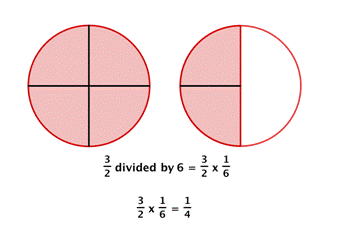Each person gets one piece, so each person gets $$\frac{1}{4}$$ of a pizza.

Dividing a fraction by a whole number is the same as multiplying by the reciprocal, so you can always use multiplication of fractions to solve division problems.

### Example

Find $$\frac{2}{3}\div 4$$.

Dividing by 4 or $$\frac{1}{4}$$.

$$\frac{2}{3}\div 4=\frac{2}{3}\cdot \frac{1}{4}$$

Multiply numerators and multiply denominators.

$$\frac{2\cdot 1}{3\cdot 4}=\frac{2}{12}$$

Simplify to lowest terms by dividing numerator and denominator by the common factor 4.

$$\frac{1}{6}$$

$$\frac{2}{3}\div4=\frac{1}{6}$$

### Example

Divide. $$9\div\frac{1}{2}$$.

Dividing by $$\frac{1}{2}$$, which is $$\frac{2}{1}$$.

$$9\div\frac{1}{2}=\frac{9}{1}\cdot\frac{2}{1}$$

Multiply numerators and multiply denominators.

$$\frac{9\cdot 2}{1\cdot 1}=\frac{18}{1}=18$$

$$9\div\frac{1}{2}=18$$

## Divide a Fraction by a Fraction

Sometimes you need to solve a problem that requires dividing by a fraction. Suppose you have a pizza that is already cut into 4 slices. How many $$\frac{1}{2}$$ slices are there?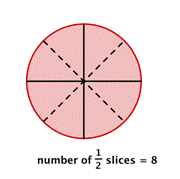There are 8 slices. You can see that dividing 4 by $$\frac{1}{2}$$ gives the same result as multiplying 4 by 2.

What would happen if you needed to divide each slice into thirds?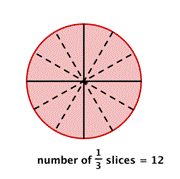You would have 12 slices, which is the same as multiplying 4 by 3.

### Dividing with Fractions

1. Find the reciprocal of the number that follows the division symbol.
2. Multiply the first number (the one before the division symbol) by the reciprocal of the second number (the one after the division symbol).

Any easy way to remember how to divide fractions is the phrase “keep, change, flip.” This means to KEEP the first number, CHANGE the division sign to multiplication, and then FLIP (use the reciprocal) of the second number.

### Example

Divide $$\frac{2}{3}\div \frac{1}{6}$$.

KEEP $$\frac{2}{3}$$

CHANGE $$\cdot$$

FLIP $$\frac{1}{6}$$

$$\frac{2}{3}\cdot \frac{6}{1}$$

Multiply numerators and multiply denominators.

$$\frac{2\cdot6}{3\cdot1}=\frac{12}{3}$$

Simplify.

$$\frac{12}{3}=4$$

$$\frac{2}{3}\div \frac{1}{6}=4$$

### Example

Divide $$\frac{3}{5}\div \frac{2}{3}$$.

[hidden-answer a=”950670″]Multiply by the reciprocal. Keep $$\div$$ to $$\frac{2}{3}$$.

$$\frac{3}{5}\cdot \frac{3}{2}$$

Multiply numerators and multiply denominators.

$$\frac{3\cdot 3}{5\cdot 2}=\frac{9}{10}$$

## Why review topics from earlier math classes?Leftovers again?

You have been in class all day, running around, picking up your kids from daycare and after a long, brutal commute you get home to realize you haven’t thought about what to fix for dinner. You go to the fridge and all you see are leftovers, a jumble of unrelated bits and pieces from meals past. Despite your fatigue, you manage to cobble together a meal that everyone will eat willingly and to get on with your evening.

In this section, you may feel like the topics are a cobbled-together meal of leftovers. The goal is to give you a reminder of some of the skills and topics from earlier math classes that, like the box of leftovers in the back of your fridge, are easily forgotten by most people. Because sometimes we just forget how these concepts work . . . until we need them again.

### Learning Outcomes

Properties of Real Numbers

• Define real numbers and subsets of real numbers
• Define properties of real numbers and sue them to evaluate algebraic expressions

Multi-Step Equations

• Use properties of real numbers to solve multi-step linear equations
• Define and use the distributive property to solve linear equations
• Classify solutions to equations

Problem Solving

• Set up equations from descriptions of problems
• Use formulas to solve application problems

As you work through the rest of the sections of this course, please return to this review if you feel like you need a reminder of the topics covered. These topics were chosen because they are often forgotten and are widely used throughout the course. Don’t worry, just like ketchup, these concepts have a long shelf life.